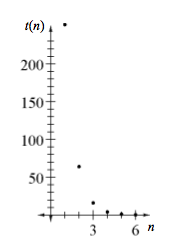### Home > A2C > Chapter 4 > Lesson 4.1.1 > Problem4-8

4-8.
1. Consider the sequence with the initial value 256, followed by 64, 16, … Homework Help ✎

1. Write the next three terms of this sequence, then find a rule for the sequence.

2. If you were to keep writing out more and more terms of the sequence, what would happen to the terms?

3. Sketch a graph of the sequence. What happens to the points as you go farther to the right?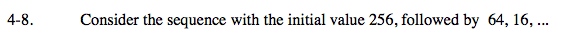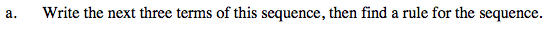Note that each number is one-fourth of the preceding number.

4, 1, 0.25

$\text{The equation is } t(n) = 1024(0.25)^{n}.$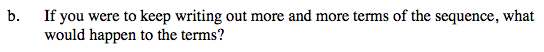As you write more terms, do they get smaller or larger? Do they ever become negative?

They get smaller, but never reach zero or become negative.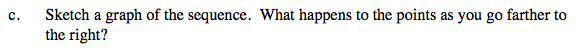Which y-value do the numbers approach?